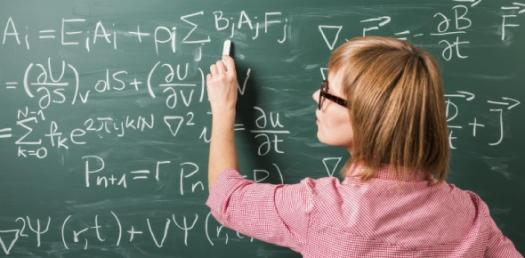# Quiz: What Do You Know About Mathematics?

10 Questions | Total Attempts: 891932SettingsMath is all around us, in everything we do. It is the building block for everything in our daily lives, including mobile devices, architecture, art, money, engineering, and even sports. Know math? Try this quiz

• 1.
Mathematics is the study of topics such as quantity, structure, space, and change.
• A.

True ..

• B.

False

• C.

Only quantity

• D.

Only Space

• 2.
Mathematics is the science that deals with the logic of shape, quantity and arrangement.
• A.

Yes, I agree

• B.

Nope, I don’t agree

• C.

It deals with only the logic of shape

• D.

It deals with only the logic of arrangement

• 3.
The word mathematics originated from?
• A.

The Mayans

• B.

The Hindus

• C.

The Jews

• D.

The Greek

• 4.
Which of these men defined mathematics as the science of quantity?
• A.

Drake

• B.

Aristotle

• C.

Pythagoras

• D.

None of the above

• 5.
One of these is NOT a type of definition of mathematics?
• A.

Logicist

• B.

Intuitionist

• C.

Formalist

• D.

None of the above

• 6.
Who was the inventor of algebra?
• A.

• B.

Carl Friedrich Gauss

• C.

Marcus du Sautoy

• D.

It invented itself

• 7.
Solve this; 0 x 1 x 2 x 3 + 4 = ?
• A.

1

• B.

2

• C.

3

• D.

4

• 8.
The square -root of 69 is?
• A.

6.3

• B.

4.3

• C.

8.3

• D.

3.3

• 9.
A plane figure with three straight sides and three angles is known as?
• A.

A tripod

• B.

A triangle

• C.

A triside

• D.

All of the above

• 10.
Who is the father of mathematics?
• A.

Gaus

• B.

Pythagoras

• C.

Aristotle

• D.

None of the above

Related TopicsBack to top